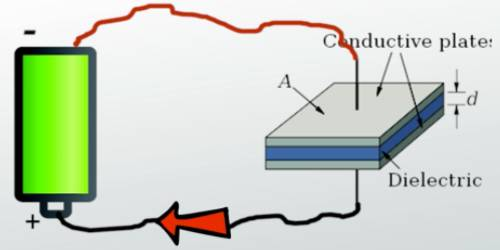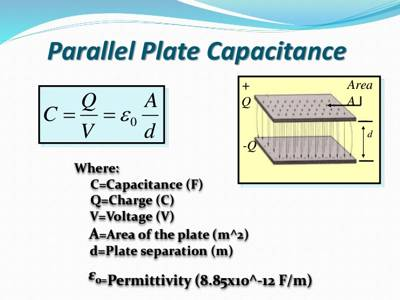Physics

# CapacitanceCapacitance

If water is poured into a container water level increases to a fixed amount. Similarly, if charges are added to a conductor its potential increases to a fixed amount. Increase in water level is proportional to the amount of water poured in; similarly, increase in the potential of a conductor is proportional to the amount of charge given to it.

Capacitance is the ability to store electrical energy. Almost all things, including you, can store some electrical energy and therefore have capacitance. When you rub your feet across a carpet, charged particles called electrons can be transferred from the carpet to you.

Suppose due to the addition of q amount of charge in a conductor its potential increases by V. Magnitude of V depends on the shape, volume, nature of the surrounding medium, and its location with respect to other conductors nearby. Besides, it depends on whether nearby conductors are isolated or connected to the ground or not.From the above discussion, it can be written,

q ∞ V

or, q/V = constant = Capacitance, or, C = q/V.

This constant is called Capacitance or capacity of the conductor. That means the amount of charge needed to increase the capacitance of a conductor by unity is called capacitance of that conductor. Its value depends on the number of charges of the conductor.

Capacitance = [Charge/Potential]